Related Articles

# Class 10 NCERT Solutions- Chapter 10 Circles – Exercise 10.2

• Difficulty Level : Easy
• Last Updated : 05 May, 2021

Theorem 10.1(NCERT) : The tangent at any point of a circle is perpendicular to the radius through the point of contact.

Theorem 10.2 (NCERT) : The lengths of tangents drawn from an external point to a circle are equal.

### Question 1. From a point Q, the length of the tangent to a circle is 24 cm and the distance of Q from the centre is 25 cm. The radius of the circle is

(A) 7 cm

(B) 12 cm

(C) 15 cm

(D) 24.5 cm

Solution: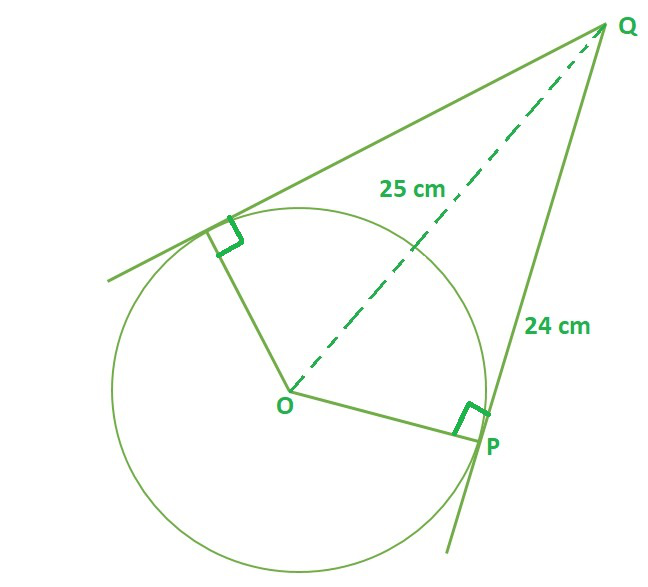According to theorem 10.1, OP⊥ PQ then ∆OPQ is a right-angled triangle

OQ2 = PQ2 + OP2  (Pythagoras Theorem)

252 = 242 + OP

OP2 = 252 – 24

OP2 = (25+24) (25-24)                               (using identity a2 – b2 = (a+b)(a-b))

OP = √49

OP = 7 cm

Hence, option A is correct.

### Question 2. In Fig. 10.11, if TP and TQ are the two tangents to a circle with centre O so that ∠ POQ = 110°, then ∠ PTQ is equal to

(A) 60°

(B) 70°

(C) 80°

(D) 90°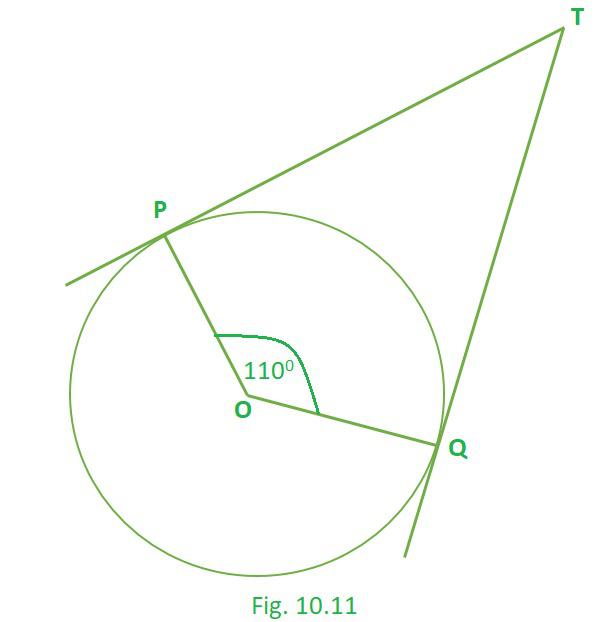Solution:

∠P = 90°, ∠Q = 90°  (Theorem 10.1)

∠O = 110°

The sum of the angles of a quadrilateral is 360° (Angle sum property of quadrilateral), Hence

∠P + ∠Q + ∠T + ∠O = 360°

90° + 90° + ∠T + 110° = 360°

∠T = 180 – 110° = 70°

Hence, option B is correct.

### Question 3. If tangents PA and PB from a point P to a circle with centre O are inclined to each other at angle of 80°, then ∠ POA is equal to

(A) 50°

(B) 60°

(C) 70°

(D) 80°

Solution: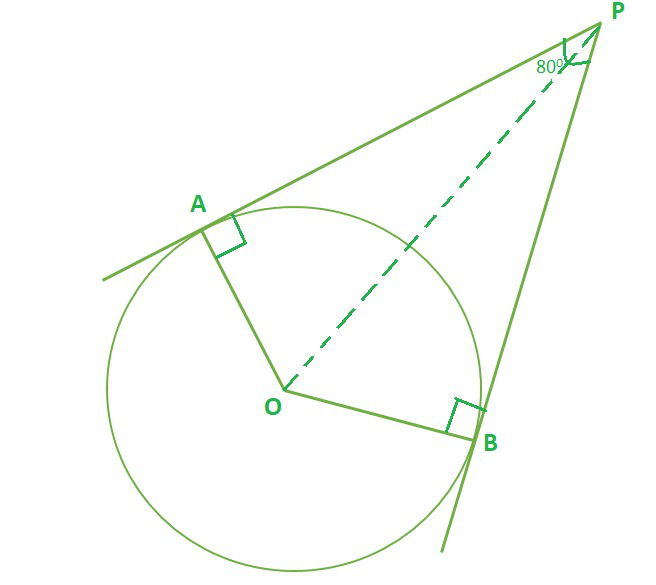∠A = 90°, ∠B = 90°  (Theorem 10.1)

∠P = 80°

The sum of the angles of a quadrilateral is 360° (Angle sum property of quadrilateral), Hence

∠A + ∠B + ∠P + ∠O = 360°

90° + 90° + 80° + ∠O = 360°

∠O = 180° – 80° = 100° …………………..(1)

Considering, ∆OAP and ∆OBP

OA = OB …………..(radius of circle)

AP = BP ……………(Theorem 10.2)

∠OAP = ∠OBP …….(Theorem 10.1)

∴ ∆OAP ≅ ∆OBP           [By SAS congruency]

So, ∠AOP = ∠BOP [By C.P.C.T.]…………..(2)

From (1) and (2), we conclude that,

∠AOP + ∠BOP = 100°

∠AOP = 50°

Hence, option A is correct.

### Question 4. Prove that the tangents drawn at the ends of a diameter of a circle are parallel.

Solution:

P and Q are point of contacts of Tangent lines l and m respectively.

O is the centre of circle.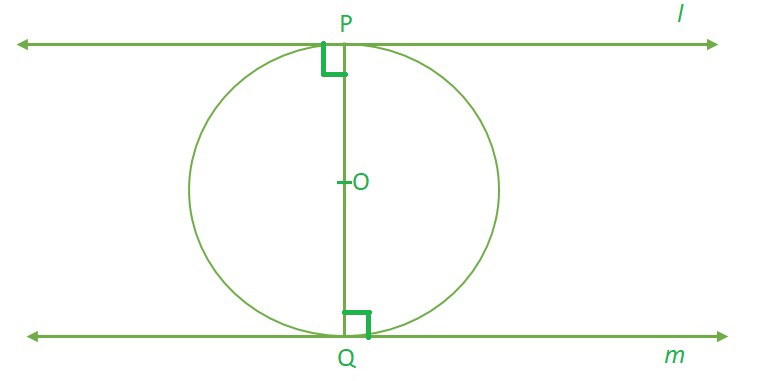OP⊥ l , OQ⊥ m and PQ is diameter (theorem 10.1)

∠PQm + ∠QPl = 90° + 90° = 180°

As, sum of adjacent angles is supplementary (180°), hence opposite sides are parallel.

### Question 5. Prove that the perpendicular at the point of contact to the tangent to a circle passes through the centre.

Solution:

P is the point of contact of tangent line l.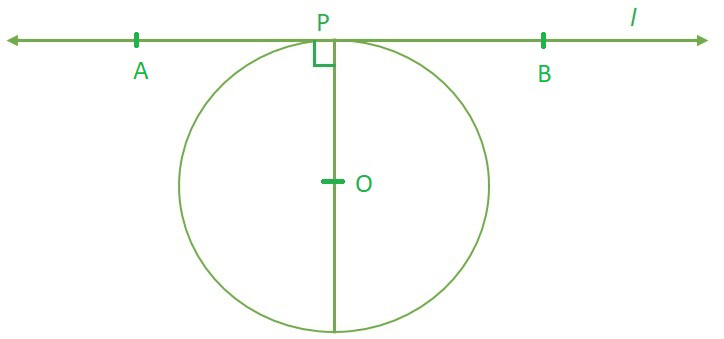Let, OP⊥ l at Point of contact P and it passes through point O.

As, The tangent at any point of a circle is perpendicular to the radius through the point of contact. (theorem 10.1)

According to the theorem 10.1 line OP has to pass through centre of circle for sure.

### Question 6. The length of a tangent from a point A at distance 5 cm from the centre of the circle is 4 cm. Find the radius of the circle.

Solution: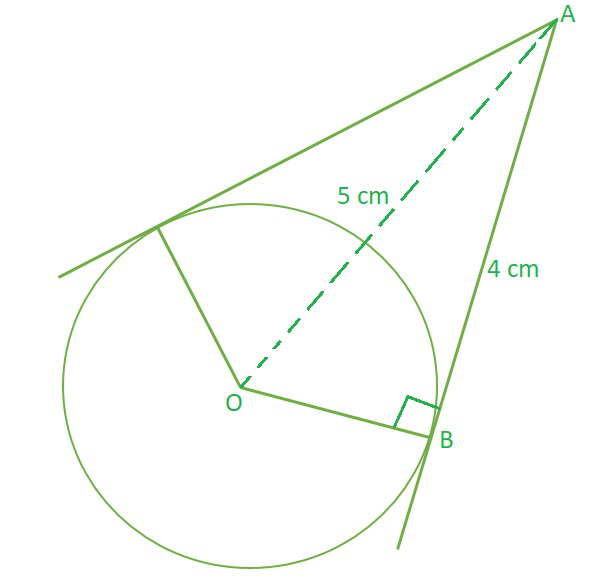According to theorem 10.1, OB⊥ AB then ∆OAB is a right-angled triangle

OA2 = AB2 + OB2  (Pythagoras Theorem)

52 = 42 + OB2

OB2 = 52 – 42

OB2 = (5+4) (5-4)                               (using identity a2 – b2 = (a+b)(a-b))

OB = √9

OB = 3 cm

Hence, Radius of circle = 3 cm

### Question 7. Two concentric circles are of radii 5 cm and 3 cm. Find the length of the chord of the larger circle which touches the smaller circle.

Solution: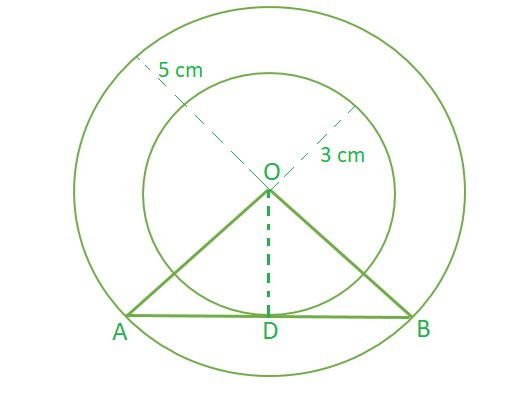OA = OB …………..(radius of large circle)

OD = OD ……………(common side)

∠ADO = ∠BDO …….(each 90°)…….(Theorem 10.1)

∴ ∆OAD ≅ ∆OBD           [By SAS congruency]

So, AD = BD [By C.P.C.T.]…………….(1)

Taking ∆OAD, which 90° at ∠D

OA = OB = 5 cm (radius of large circle)

OD = 3 cm  (radius of smaller circle)

OA2 = AD2 + OD2  (Pythagoras Theorem)

AB = 2 × AD (from 1)

AB = 2 × 4

AB = 8 cm

Hence, length of the chord of the larger circle which touches the smaller circle = 8 cm

### Question 8. A quadrilateral ABCD is drawn to circumscribe a circle (see Fig. 10.12). Prove that AB + CD = AD + BC.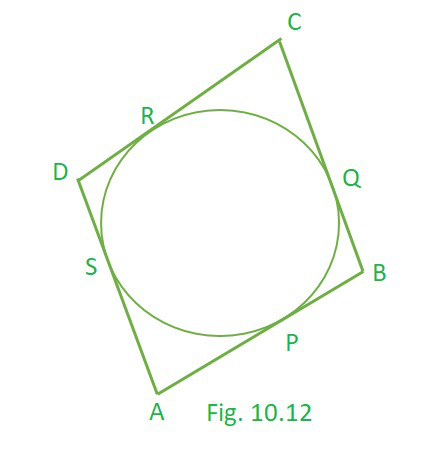Solution:

Let the P, Q, R and S be point of contacts for tangent AB, BC, CD and DA respectively .

AP = AS  (theorem 10.2)……..(1)

BP = BQ  (theorem 10.2)……..(2)

CR = CQ  (theorem 10.2)……..(3)

DR = DS  (theorem 10.2)……..(4)

By, adding (1), (2), (3) and (4) RHS = LHS, we get

AP + BP + CR + DR = AS + BQ + CQ + DS

(AP + BP) + (CR + DR) = (AS + DS) + (BQ + CQ)  (by Rearranging)

AB + CD = AD + BC

Hence, proved !!

### Question 9. In Fig. 10.13, XY and X′Y′ are two parallel tangents to a circle with centre O and another tangent AB with point of contact C intersecting XY at A and X′Y′ at B. Prove that ∠ AOB = 90°.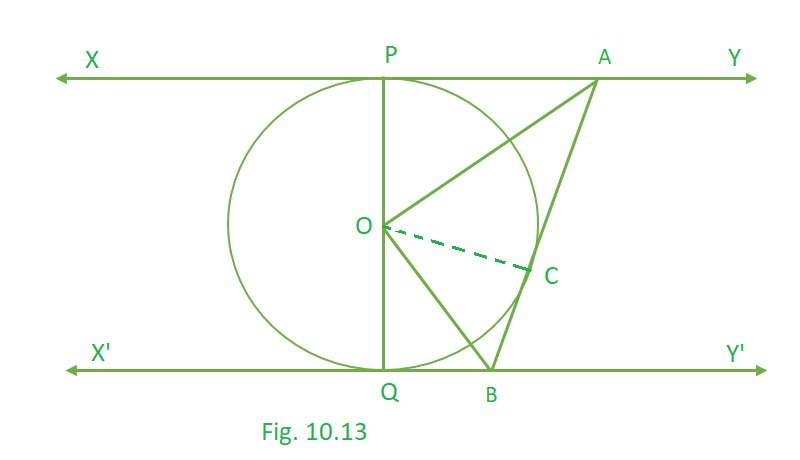Solution:

As we can observe here,

AP and AC are tangent at same external point A.

and, QB and BC are tangent at same external point B.

Taking, ∆OAP and ∆OAC in consideration

OP = OC …………..(radius of circle)

OA = OA ……………(common side)

∠OPA = ∠OCA …….(each 90°)…….(Theorem 10.1)

∴ ∆OAP ≅ ∆OAC           [By SAS congruency]

So, ∠POA = ∠COA [By C.P.C.T.]

we can conclude that, ∠COP = 2 ∠COA………………..(1)

Similarly, ∠COQ = 2 ∠COB ………….(2)

Adding (1) and (2), RHS = LHS we get,

2 ∠COA + 2 ∠COB = ∠COQ + ∠COP

2 (∠COA + ∠COB) = 180°  (Angle made by a straight line = 180°)

2 (∠AOB) = 180°

∠AOB = 90°.

Hence, proved !!

### Question 10. Prove that the angle between the two tangents drawn from an external point to a circle is supplementary to the angle subtended by the line segment joining the points of contact at the Centre.

Solution: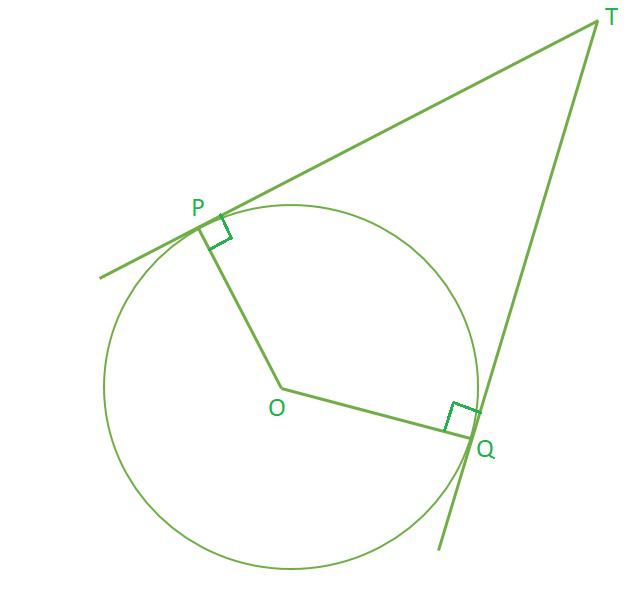∠P = 90°, ∠Q = 90°  (Theorem 10.1)

The sum of the angles of a quadrilateral is 360° (Angle sum property of quadrilateral), Hence

∠P + ∠Q + ∠T + ∠O = 360°

90° + 90° + ∠T + ∠O = 360°

∠T + ∠O = 180°

Hence, Proved, the angle between the two tangents drawn from an external point to a circle is supplementary to the angle subtended by the line segment joining the points of contact at the Centre.

### Question 11. Prove that the parallelogram circumscribing a circle is a rhombus.

Solution:

ABCD is a parallelogram and let P, Q, R and S be the point of contact of circle and parallelogram.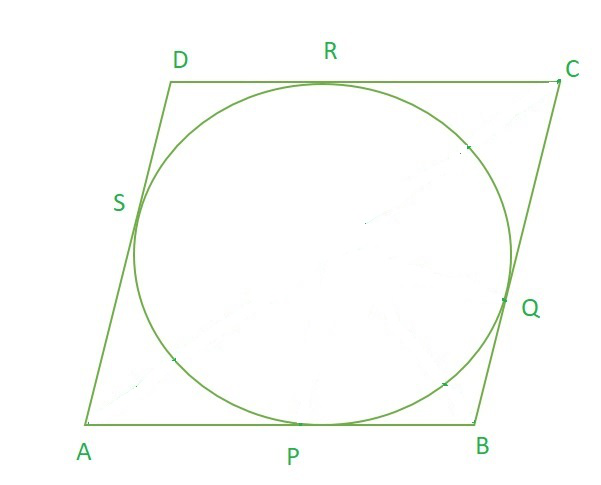AP = AS  (theorem 10.2)……..(1)

BP = BQ  (theorem 10.2)……..(2)

CR = CQ  (theorem 10.2)……..(3)

DR = DS  (theorem 10.2)……..(4)

By, adding (1), (2), (3) and (4) RHS = LHS, we get

AP + BP + CR + DR = AS + BQ + CQ + DS

(AP + BP) + (CR + DR) = (AS + DS) + (BQ + CQ)  (by Rearranging)

AB + CD = AD + BC

As ABCD is a parallelogram, AB = CD and AD = BC (Opposite sides of parallelogram are equal)

Hence, 2 AB = 2 BC

AB = BC

If adjacent side of parallelogram are equal, then it is a rhombus.

Hence, ABCD is a rhombus !!

### Question 12. A triangle ABC is drawn to circumscribe a circle of radius 4 cm such that the segments BD and DC into which BC is divided by the point of contact D are of lengths 8 cm and 6 cm respectively (see Fig. 10.14). Find the sides AB and AC.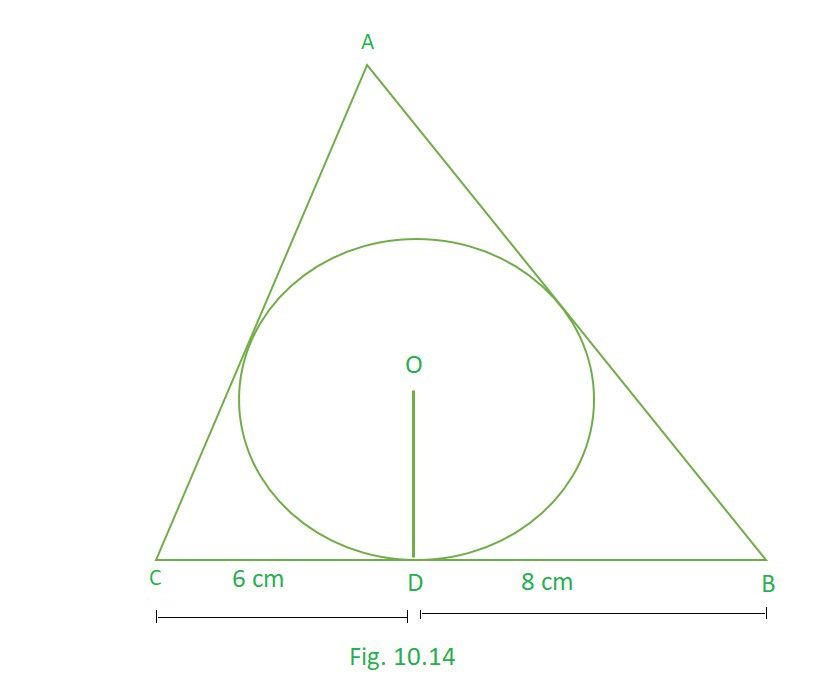Solution:

ABC is a Triangle and let M, D and N be the point of contact of circle and Triangle.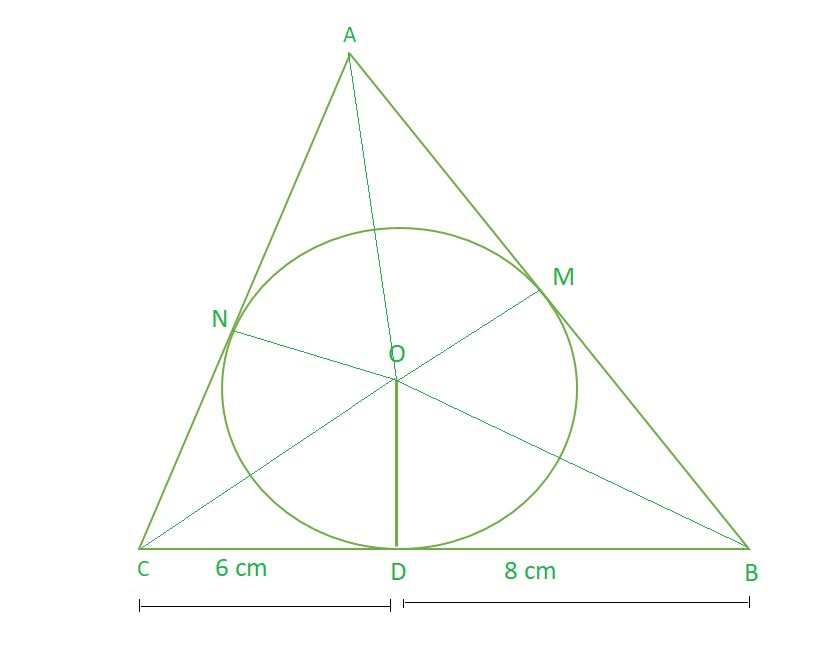BD = BM =  8 cm (theorem 10.2)……..(1)

CN = CD  = 6 cm (theorem 10.2)……..(2)

AN = AM  = p cm (theorem 10.2)……..(3)

AB = p+8 cm

BC = 6+8 = 14 cm

AC = p+6 cm

As we can observe here that,

Area of ∆ABC = Area of ∆AOC + Area of ∆COB + Area of ∆BOA

So, Area of ∆ABC = ar(∆ABC)

√(s (s-AB) (s-AC) (s-BC))     …………(Heron’s formula)  where s = (sum of sides) / 2

s = (AB+BC+AC)/2

s = (p+8+14+p+6)/2

s = (2p+28)/2

s = p+14

ar(∆ABC) = √((p+14) (p+14-(p+8)) (p+14-(p+6)) (p+14-14))

= √((p+14) (6) (8) (p))

= √48p (p+14) cm2……………………………………………(1)

Area of ∆AOC + Area of ∆COB + Area of ∆BOA = (½ × ON × AC) + (½ × OD × BC) + (½ × OM × AB)

= (½ × 4 × (p+6)) + (½ × 4 × 14) + (½ × 4 × (p+8))

= ½ × 4 (p+6+14+p+8)

= ½ × 4 × (2p+28)

= 4 × (p+14) cm2  ………………………………………………(2)

(1) = (2)

√48p (p+14) =  4 × (p+14)

Squaring both sides, we get

48 × p × (p+14) = (4 × (p+14))2

48 × p (p+14) = 16 × (p+14)2             (cancelling (p+14) from both sides)

48 × p = 16 (p+14)

48p = 16p+ 224

32 × p = 224

p = 7 cm

Hence, AB = p+8 = 7+8 = 15 cm

AC = p+6 = 7+6 = 13 cm

### Question 13. Prove that opposite sides of a quadrilateral circumscribing a circle subtends supplementary angles at the Centre of the circle.

Solution: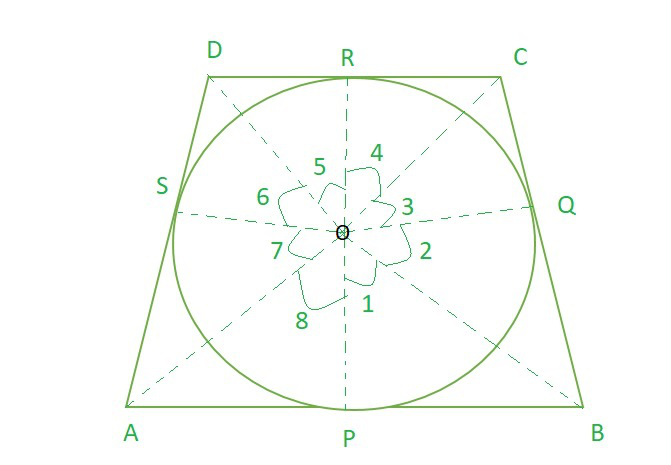∠OPB = 90° , ∠OQB = 90°  (Theorem 10.1)

Considering, ∆OPB and ∆OQB

OP = OQ …………..(radius of circle)

OB = OB ……………(Common)

∠OPB = ∠OQB …….(each 90°)……..(Theorem 10.1)

∴ ∆OPB ≅ ∆OQB           [By SAS congruency]

So, ∠POB = ∠QOB [By C.P.C.T.]

Hence, ∠1 = ∠2  ……………..(1)

Similarly, ∠3 = ∠4  ……………..(2)

∠5 = ∠6  ……………..(3)

∠7 = ∠8  ……………..(4)

By making complete revolution,

∠1 + ∠2 + ∠3 + ∠4 + ∠5 + ∠6 + ∠7 + ∠8 = 360° (A complete revolution makes 360°)

∠1 + ∠1 + ∠4 + ∠4 + ∠5 + ∠5 + ∠8 + ∠8 = 360°

2 (∠1 + ∠4 + ∠5 + ∠8) = 360°

2 ((∠1 + ∠8) + (∠4 + ∠5) ) = 360°

∠AOB + ∠COD = 360° / 2 = 180°

Hence, proved, that opposite sides of a quadrilateral circumscribing a circle subtend supplementary angles at the Centre of the circle.

Attention reader! All those who say programming isn’t for kids, just haven’t met the right mentors yet. Join the  Demo Class for First Step to Coding Coursespecifically designed for students of class 8 to 12.

The students will get to learn more about the world of programming in these free classes which will definitely help them in making a wise career choice in the future.

My Personal Notes arrow_drop_up